2022 Norris Trophy Winner Odds
-320
0.31 to 1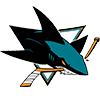Erik Karlsson
65.2% implied probability

+900
9 to 1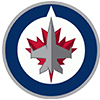Josh Morrissey
8.6% implied probability

+1000
10 to 1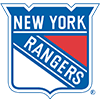Adam Fox
7.8% implied probability

+1200
12 to 1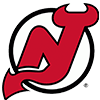Dougie Hamilton
6.6% implied probability

+1200
12 to 1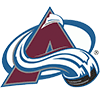Cale Makar
6.6% implied probability

+5000
50 to 1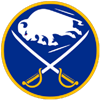Rasmus Dahlin
1.7% implied probability

+10000
100 to 1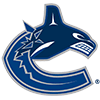Quinn Hughes
0.8% implied probability

+15000
150 to 1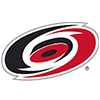Brent Burns
0.6% implied probability

+15000
150 to 1Roman Josi
0.6% implied probability

+15000
150 to 1Charlie McAvoy
0.6% implied probability

+15000
150 to 1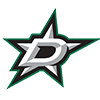Miro Heiskanen
0.6% implied probability

+30000
300 to 1Hampus Lindholm
0.3% implied probability

+50000
500 to 1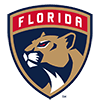Brandon Montour
0.2% implied probability